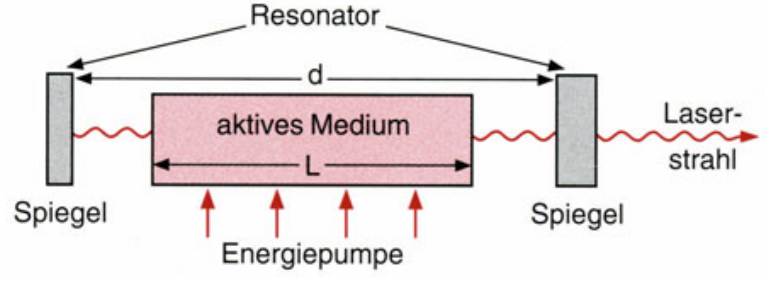This calculator will help you computes the round trip time and repetition frequency of a pulsed laser cavity.### $f_{rep}$= $\frac{1}{\tau}$

Material 1 (or2) length with double pass, L$_1$ (L$_2$);

Material 3 (or4) length with single pass, L$_3$ (L$_4$);

Material 1 (2,3,4) group index, $n_{g^1} (n_{g^2} n_{g^3} n_{g^4})$;

Round Trip Time, $\tau$    Repetition Frequency, f$_{rep}$

Input
m
m
m
m

Output
ns
MHz